# Machine Learning Using Python (MEAFA Workshop)

## Lesson 2: Regularised Linear Models

This lesson introduces regularisation, one of the most important ideas in machine learning.

This notebook relies on the following libraries and settings.

In :
# Packages
import numpy as np
from scipy import stats
import pandas as pd
import matplotlib.pyplot as plt
import seaborn as sns
import warnings
warnings.filterwarnings('ignore')

In :
# Plot settings
sns.set_context('notebook')
sns.set_style('ticks')
colours = ['#1F77B4', '#FF7F0E', '#2CA02C', '#DB2728', '#9467BD', '#8C564B', '#E377C2','#7F7F7F', '#BCBD22', '#17BECF']
crayon = ['#4E79A7','#F28E2C','#E15759','#76B7B2','#59A14F', '#EDC949','#AF7AA1','#FF9DA7','#9C755F','#BAB0AB']
sns.set_palette(colours)
%matplotlib inline
plt.rcParams['figure.figsize'] = (9, 6)

In :
# Model selection and evaluation tools
from sklearn.model_selection import train_test_split
from sklearn.model_selection import RandomizedSearchCV
from sklearn.metrics import mean_squared_error, r2_score,  mean_absolute_error

# Methods
from sklearn.linear_model import LinearRegression


## House Pricing Data¶

We use data from De Cock (2011), who compiled a detailed dataset of residential property sales in a North American city. It is used in one of the most popular practice Kaggle competitions. This dataset is characterised by a large number of predictor variables (81, including categorical, ordinal, discrete, and continuous variables). See the documentation for a description of the original variables.

I cleaned and processed the original dataset into a version that is ready analysis. The dataset has 196 variables after coding the categorical predictors using dummy variables and the creation of other relevant variables.

In :
data=pd.read_csv('Datasets/AmesHousing-Processed.csv')

Out:
1stFlrSF 2ndFlrSF 3SsnPorch Age BsmtFinSF1 BsmtFinSF2 BsmtUnfSF EnclosedPorch GarageArea LotArea ... RoofMatl_Other RoofStyle_Hip RoofStyle_Other ScreenPorchZero WoodDeckSFZero YrSold_2007 YrSold_2008 YrSold_2009 YrSold_2010 SalePrice
0 1656 0 0 50 639.0 0.0 441.0 0 528.0 31770 ... 0 1 0 1 0 0 0 0 1 215000
1 896 0 0 49 468.0 144.0 270.0 0 730.0 11622 ... 0 0 0 0 0 0 0 0 1 105000
2 1329 0 0 52 923.0 0.0 406.0 0 312.0 14267 ... 0 1 0 1 0 0 0 0 1 172000
3 2110 0 0 42 1065.0 0.0 1045.0 0 522.0 11160 ... 0 1 0 1 1 0 0 0 1 244000
4 928 701 0 13 791.0 0.0 137.0 0 482.0 13830 ... 0 0 0 1 0 0 0 0 1 189900

5 rows × 196 columns

We the split the data into training and test sets. We use a small training dataset to better illustrate the advantages of regularisation.

In :
response='SalePrice'
predictors=list(data.columns.values[:-1])

# Randomly split indexes
index_train, index_test  = train_test_split(np.array(data.index), train_size=0.2, random_state=5)

# Write training and test sets
train = data.loc[index_train,:].copy()
test =  data.loc[index_test,:].copy()

y_train = train[response]
y_test = test[response]

X_train = train[predictors].copy()
X_test = test[predictors].copy()


## Exploratory data analysis¶

In :
from statlearning import plot_histogram

plot_histogram(y_train)
plt.title('Distribution of Sale Prices')
plt.show()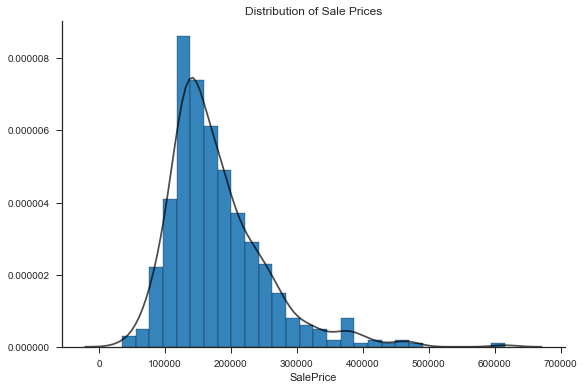Many of the numerical variables have a substantial number of zeros, since they refer to variables that do not apply to all houses (such as the second floor area). The dataset includes additional constructed features to account for this feature of the data.

In :
from statlearning import plot_regressions

with sns.color_palette(crayon):
plot_regressions(X_train.iloc[:,:21], y_train)
plt.show()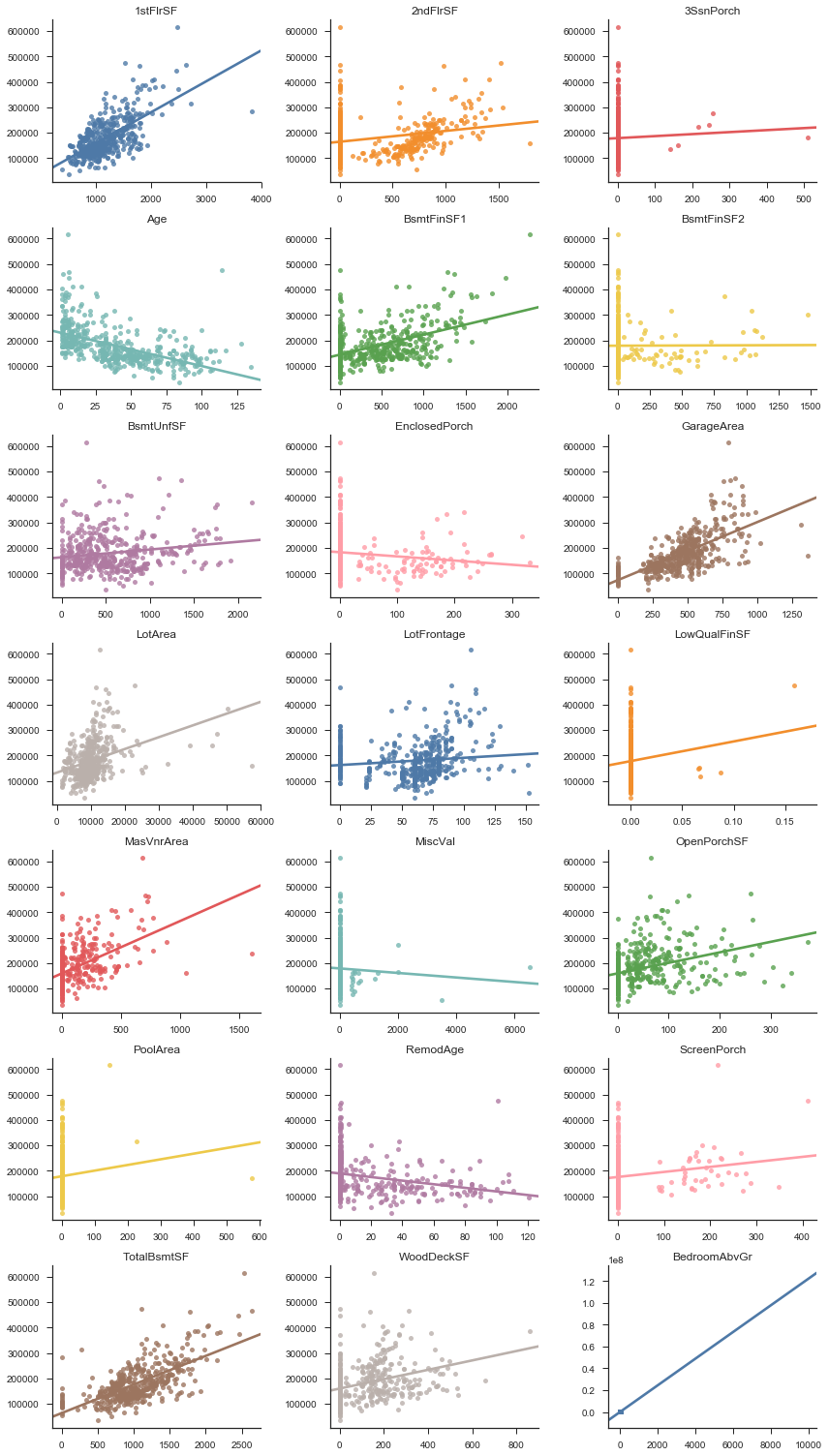## Response Transformation¶

The above plots show that (i) the response variable is right skewed (ii) there are nonlinear relationships between the response and certain predictors (iii) the noise has non-constant variance as a function of the predictors (see for example the funnel shape in the first scatter plot).

It is very common to observe these three patterns together in economic data, for reasons such as diminishing returns (additional space becomes less valuable if you have more space to begin with) and inflation (prices, salaries, etc, increase in percentage terms, not in a linear fashion).

In this situation, transforming the response will typically improve performance, as it is a parsimonious way to account for nonlinearities, reduce skewness, and make the noise variance near constant. The log transformation is the most common transformation of this type.

In :
y_train = np.log(train[response])
y_test = np.log(test[response])


Compare the univariate plots below with the original.

In :
from statlearning import plot_regressions

with sns.color_palette(crayon):
plot_regressions(X_train.iloc[:,:6], y_train)
plt.show()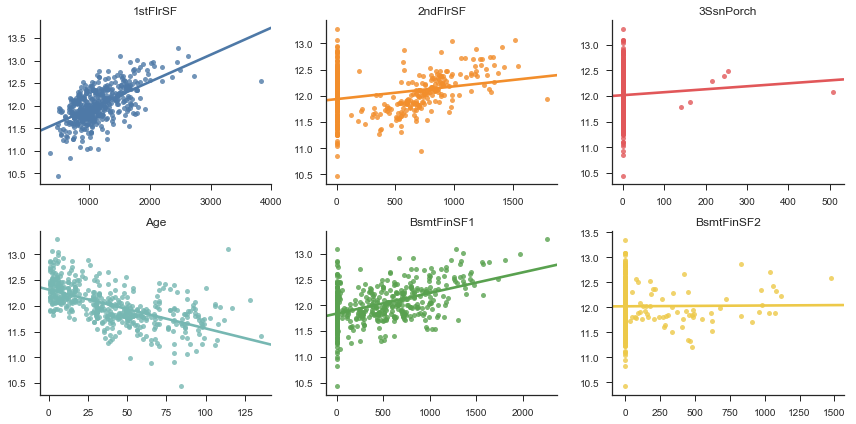## Scaling the Predictors¶

It is essential to scale the predictors before fitting regularised linear methods. Like several other machine learning algorithms, regularised linear methods do not perform well when predictors have very different scales (because the penalty term in the objective function for training treats all coefficients as comparable).

The most common type of scaling is standardisation, where we transform each predictor by subtracting its sample average and diving the result by its standard deviation. The Scikit-Learn StandardScaler method makes it easy to process the data in this way.

We have two ways of implementing a workflow that includes scaling. The first is to use the standard scaler to preprocess the design matrix, and then work with the transformed as usual.

In :
from sklearn.preprocessing import StandardScaler

scaler = StandardScaler()
scaler.fit(X_train)

X_train_scaled = scaler.transform(X_train)
X_test_scaled = scaler.transform(X_test)


We use a ridge regression to illustrate the process of fitting the model and computing predictions, without worrying about the details of the algorithm for now.

In :
from sklearn.linear_model import Ridge

ridge = Ridge()
ridge.fit(X_train_scaled, y_train)
y_pred = ridge.predict(X_test_scaled)
print(y_pred) # we will get the result below

11.2982722241


The other option is to use a Scikit-Learn pipeline, which allows the user to combine transformations with a final estimator.

In :
from sklearn.pipeline import Pipeline

ridge = Pipeline([
('scaler', StandardScaler()),
('estimator', Ridge()),
])

ridge.fit(X_train, y_train)
y_pred = ridge.predict(X_test)
print(y_pred)

11.2982722241


## Ridge Regression¶

In :
from sklearn.linear_model import RidgeCV

alphas = list(np.logspace(-15, 15, 151, base=2))

ridge = RidgeCV(alphas=alphas, cv=5)
ridge.fit(X_train_scaled, y_train)

Out:
RidgeCV(alphas=[3.0517578125e-05, 3.5055491790680982e-05, 4.0268185753567341e-05, 4.6255998733837822e-05, 5.3134189654304478e-05, 6.103515625e-05, 7.0110983581361965e-05, 8.0536371507134683e-05, 9.2511997467675645e-05, 0.00010626837930860896, 0.0001220703125, 0.00014022196716272393, 0.00016107274301426937, ...05, 16384.0, 18820.273848271459, 21618.817610103131, 24833.500225706386, 28526.20085808741, 32768.0],
cv=5, fit_intercept=True, gcv_mode=None, normalize=False, scoring=None,
store_cv_values=False)
In :
ridge = Pipeline((
('scaler', StandardScaler()),
('estimator', RidgeCV(alphas=alphas, cv=5)),
))

ridge.fit(X_train, y_train)

Out:
Pipeline(memory=None,
steps=[('scaler', StandardScaler(copy=True, with_mean=True, with_std=True)), ('estimator', RidgeCV(alphas=[3.0517578125e-05, 3.5055491790680982e-05, 4.0268185753567341e-05, 4.6255998733837822e-05, 5.3134189654304478e-05, 6.103515625e-05, 7.0110983581361965e-05, 8.0536371507134683e-05, 9.251199746767...cv=5, fit_intercept=True, gcv_mode=None, normalize=False, scoring=None,
store_cv_values=False))])
In :
from statlearning import plot_coefficients
plot_coefficients(ridge._final_estimator, predictors)
plt.show()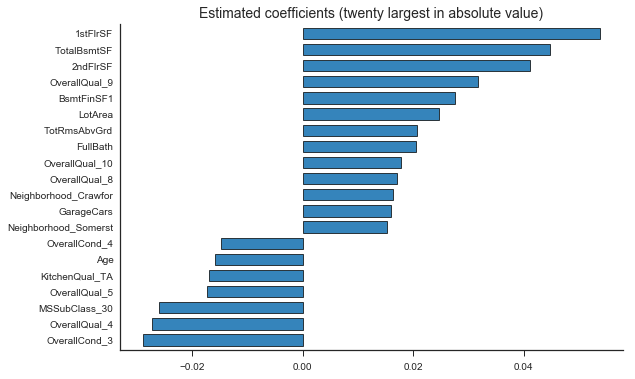## Lasso¶

We use the LassoCV class to fit and tune the lasso model. The reason for this class, as opposed to running a grid search on the Lasso class, is that there is an efficient algorithm for computing the path of solutions as we vary the lasso penalty. The same is true of other models.

Below, we present code according to the two ways of handling predictor scaling.

In :
from sklearn.linear_model import LassoCV

lasso = LassoCV(cv=5)
lasso.fit(X_train_scaled, y_train)

Out:
LassoCV(alphas=None, copy_X=True, cv=5, eps=0.001, fit_intercept=True,
max_iter=1000, n_alphas=100, n_jobs=1, normalize=False, positive=False,
precompute='auto', random_state=None, selection='cyclic', tol=0.0001,
verbose=False)

Now, using a pipeline.

In :
lasso = Pipeline((
('scaler', StandardScaler()),
('estimator', LassoCV(cv=5)),
))

lasso.fit(X_train, y_train)

Out:
Pipeline(memory=None,
steps=[('scaler', StandardScaler(copy=True, with_mean=True, with_std=True)), ('estimator', LassoCV(alphas=None, copy_X=True, cv=5, eps=0.001, fit_intercept=True,
max_iter=1000, n_alphas=100, n_jobs=1, normalize=False, positive=False,
precompute='auto', random_state=None, selection='cyclic', tol=0.0001,
verbose=False))])

The lasso shrinks 88 coefficients to zero.

In :
reg = lasso._final_estimator # the final estimator attribute refers to the pipeline
np.sum(reg.coef_==0.0)

Out:
88
In :
from statlearning import plot_coefficients

plot_coefficients(reg, predictors)
plt.show()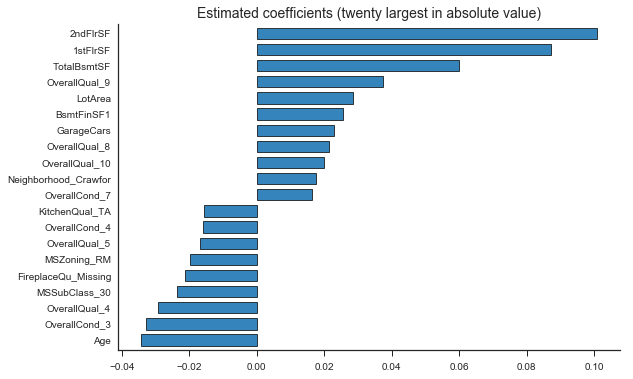## Elastic Net¶

In :
from sklearn.linear_model import ElasticNetCV

enet = ElasticNetCV(l1_ratio=[0.01,0.1,0.2,0.3,0.4,0.5,0.6,0.7,0.8,0.9, 0.99], cv=5)
enet.fit(X_train_scaled, y_train)

Out:
ElasticNetCV(alphas=None, copy_X=True, cv=5, eps=0.001, fit_intercept=True,
l1_ratio=[0.01, 0.1, 0.2, 0.3, 0.4, 0.5, 0.6, 0.7, 0.8, 0.9, 0.99],
max_iter=1000, n_alphas=100, n_jobs=1, normalize=False,
positive=False, precompute='auto', random_state=None,
selection='cyclic', tol=0.0001, verbose=0)
In :
enet.l1_ratio_

Out:
0.98999999999999999
In :
enet = Pipeline((
('scaler', StandardScaler()),
('estimator', ElasticNetCV(l1_ratio=[0.01,0.1,0.2,0.3,0.4,0.5,0.6,0.7,0.8,0.9, 0.99], cv=5, n_alphas=200)),
))

enet.fit(X_train, y_train)

Out:
Pipeline(memory=None,
steps=[('scaler', StandardScaler(copy=True, with_mean=True, with_std=True)), ('estimator', ElasticNetCV(alphas=None, copy_X=True, cv=5, eps=0.001, fit_intercept=True,
l1_ratio=[0.01, 0.1, 0.2, 0.3, 0.4, 0.5, 0.6, 0.7, 0.8, 0.9, 0.99],
max_iter=1000, n_alphas=200, n_jobs=1, normalize=False,
positive=False, precompute='auto', random_state=None,
selection='cyclic', tol=0.0001, verbose=0))])
In :
plot_coefficients(enet._final_estimator, predictors)
plt.show()## Model Evaluation¶

We compute the test results for the original price scale.

As a technical detail, we should make a bias correction when predicting the original response based on a model for a transformed response. However, the adjustment is very small for the current example (see below), so that we ignore it for simplicity.

In :
ols = LinearRegression()
ols.fit(X_train, y_train)

columns=['Test RMSE', 'Test R2', 'Test MAE']
rows=['OLS', 'Lasso', 'Ridge', 'Elastic Net']
results=pd.DataFrame(0.0, columns=columns, index=rows)

methods=[ols, lasso, ridge, enet]

for i, method in enumerate(methods):

y_pred=method.predict(X_test)
results.iloc[i,0] = np.sqrt(mean_squared_error(np.exp(y_test), np.exp(y_pred)))
results.iloc[i,1] = r2_score(np.exp(y_test), np.exp(y_pred))
results.iloc[i,2] = mean_absolute_error(np.exp(y_test), np.exp(y_pred))

results.round(3)

Out:
Test RMSE Test R2 Test MAE
OLS 31516.366 0.792 16134.551
Lasso 21064.675 0.907 13028.499
Ridge 21780.871 0.901 14008.729
Elastic Net 21057.507 0.907 13025.408
In :
y_fit = lasso.predict(X_train)
np.mean(np.exp(y_train-y_fit))   # leave-one-out residuals are better if convenient to compute

Out:
1.0026020179685708

The code for computing the test predictions using this method is the following.

In :
y_pred = lasso.predict(X_test)
y_pred = y_pred*np.mean(np.exp(y_train-y_fit)) # the correction is based on the training data!